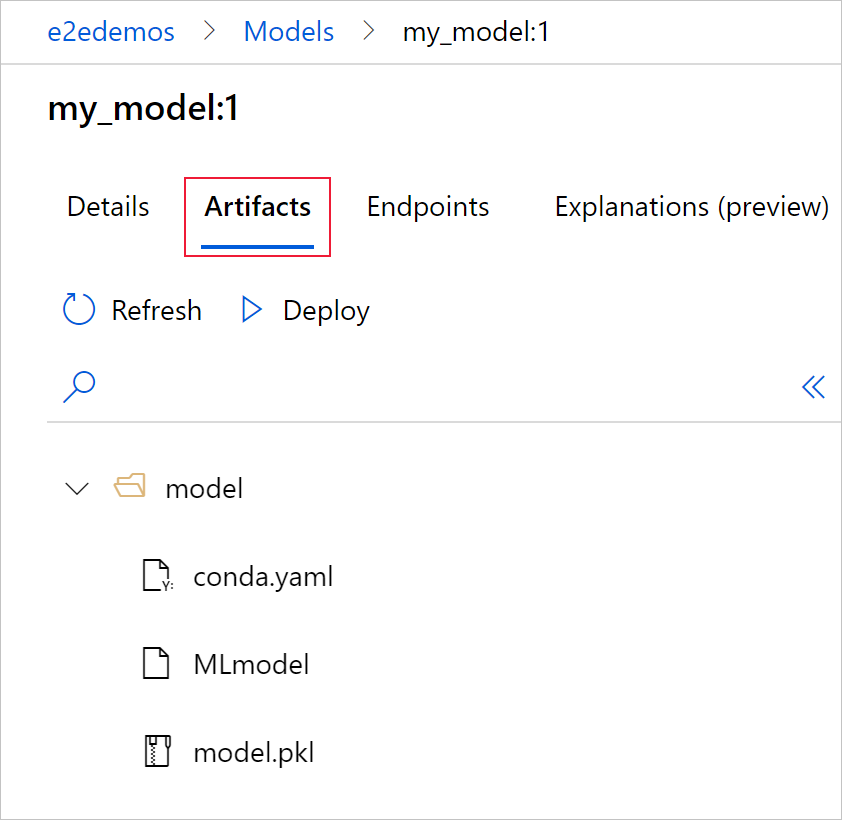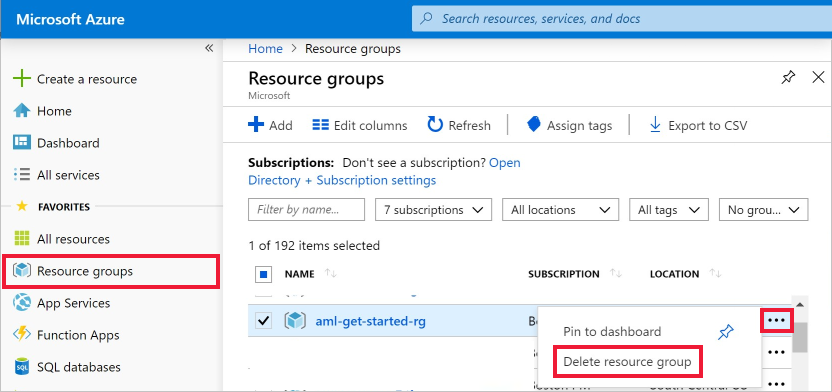# 使用 MLflow 和 Azure 机器学习跟踪 ML 模型

MLflow 是一个开放源代码库，用于管理机器学习试验的生命周期。 MLFlow 跟踪是 MLflow 的一个组件，可以记录和跟踪训练运行指标和模型项目，而不管试验环境是位于本地计算机、远程计算目标、虚拟机还是 Azure Databricks 群集上。

## 先决条件

• 安装 `mlflow` 包。

• 可以使用 MLflow Skinny，它是一个不带 SQL 存储、服务器、UI 或数据科学依赖项的轻型 MLflow 包。 对于主要需要用到跟踪和日志记录功能，但不需要导入整个 MLflow 功能套件（包括部署）的用户，建议使用此客户端。
• 安装 `azureml-mlflow` 包。

• 安装并设置 Azure 机器学习 CLI (v1)，并确保安装 ml 扩展。

重要

本文中的一些 Azure CLI 命令使用适用于 Azure 机器学习的 `azure-cli-ml` 或 v1 扩展。 对 v1 扩展的支持将于 2025 年 9 月 30 日结束。 在该日期之前，你将能够安装和使用 v1 扩展。

建议在 2025 年 9 月 30 日之前转换为 `ml` 或 v2 扩展。 有关 v2 扩展的详细信息，请参阅 Azure ML CLI 扩展和 Python SDK v2

## 从本地计算机或远程计算跟踪运行

### 设置跟踪环境

1. 使用工作区配置文件：

``````from azureml.core import Workspace
import mlflow

ws = Workspace.from_config()
mlflow.set_tracking_uri(ws.get_mlflow_tracking_uri())
``````

提示

可以通过以下方式下载工作区配置文件：

1. 导航到 Azure ML 工作室
2. 单击页面右上角 -> 下载配置文件。
3. 将文件 `config.json` 保存到正在使用的同一目录中。
2. 使用订阅 ID、资源组名称和工作区名称：

``````from azureml.core import Workspace
import mlflow

#Enter details of your AzureML workspace
subscription_id = '<SUBSCRIPTION_ID>'
resource_group = '<RESOURCE_GROUP>'
workspace_name = '<AZUREML_WORKSPACE_NAME>'

ws = Workspace.get(name=workspace_name,
subscription_id=subscription_id,
resource_group=resource_group)

mlflow.set_tracking_uri(ws.get_mlflow_tracking_uri())
``````

### 设置试验名称

``````experiment_name = 'experiment_with_mlflow'
mlflow.set_experiment(experiment_name)
``````

### 启动训练运行

``````import os
from random import random

with mlflow.start_run() as mlflow_run:
mlflow.log_param("hello_param", "world")
mlflow.log_metric("hello_metric", random())
os.system(f"echo 'hello world' > helloworld.txt")
mlflow.log_artifact("helloworld.txt")
``````

## 跟踪 Azure 机器学习中运行的作业

### 创建训练例程

``````# imports
import os
import mlflow

from random import random

# define functions
def main():
mlflow.log_param("hello_param", "world")
mlflow.log_metric("hello_metric", random())
os.system(f"echo 'hello world' > helloworld.txt")
mlflow.log_artifact("helloworld.txt")

# run functions
if __name__ == "__main__":
# run main function
main()
``````

### 配置试验

``````from azureml.core import Environment

env = Environment(name="mlflow-env")

# Specify conda dependencies with scikit-learn and temporary pointers to mlflow extensions
conda_packages=["scikit-learn", "matplotlib"],
pip_packages=["azureml-mlflow", "pandas", "numpy"]
)

env.python.conda_dependencies = cd
``````

``````from azureml.core import ScriptRunConfig

src = ScriptRunConfig(source_directory="src",
script=training_script,
compute_target="<COMPUTE_NAME>",
environment=env)
``````

``````from azureml.core import Experiment
from azureml.core import Workspace
ws = Workspace.from_config()

experiment_name = "experiment_with_mlflow"
exp = Experiment(workspace=ws, name=experiment_name)

run = exp.submit(src)
``````

## 查看工作区中的指标和项目

``````from mlflow.tracking import MlflowClient

# Use MlFlow to retrieve the run that was just completed
client = MlflowClient()
run_id = mlflow_run.info.run_id
finished_mlflow_run = MlflowClient().get_run(run_id)

metrics = finished_mlflow_run.data.metrics
tags = finished_mlflow_run.data.tags
params = finished_mlflow_run.data.params

print(metrics,tags,params)
``````

``````client.list_artifacts(run_id)
``````

``````client.download_artifacts(run_id, "helloworld.txt", ".")
``````

## 比较和查询

``````from mlflow.entities import ViewType

all_experiments = [exp.experiment_id for exp in MlflowClient().list_experiments()]
query = "metrics.hello_metric > 0"
runs = mlflow.search_runs(experiment_ids=all_experiments, filter_string=query, run_view_type=ViewType.ALL)

``````

## 自动日志记录

``````mlflow.autolog()
``````

## 管理模型

1. 运行完成后，调用 `register_model()` 方法。

``````# the model folder produced from a run is registered. This includes the MLmodel file, model.pkl and the conda.yaml.
model_path = "model"
model_uri = 'runs:/{}/{}'.format(run_id, model_path)
mlflow.register_model(model_uri,"registered_model_name")
``````
2. 使用 Azure 机器学习工作室查看工作区中的已注册模型。

在以下示例中，已注册的模型 `my-model` 标记了 MLflow 跟踪元数据。3. 选择“项目”选项卡以查看与 MLflow 模型架构（conda.yaml、MLmodel 和 model.pkl）一致的所有模型文件。4. 选择 MLmodel 以查看运行生成的 MLmodel 文件。## 清理资源

1. 在 Azure 门户中，选择最左侧的“资源组”。2. 从列表中选择已创建的资源组。

3. 选择“删除资源组”

4. 输入资源组名称。 然后选择“删除”。